gemseo / post / dataset

# scatter_plot_matrix module¶

Draw a scatter matrix from a Dataset.

The ScatterMatrix class implements the scatter plot matrix, which is a way to visualize $$n$$ samples of a multi-dimensional vector

$x=(x_1,x_2,\ldots,x_d)\in\mathbb{R}^d$

in several 2D subplots where the (i,j) subplot represents the cloud of points

$\left(x_i^{(k)},x_j^{(k)}\right)_{1\leq k \leq n}$

while the (i,i) subplot represents the empirical distribution of the samples

$x_i^{(1)},\ldots,x_i^{(n)}$

by means of an histogram or a kernel density estimator.

A variable name can be passed to the DatasetPlot.execute() method by means of the classifier keyword in order to color the curves according to the value of the variable name. This is useful when the data is labeled.

class gemseo.post.dataset.scatter_plot_matrix.ScatterMatrix(dataset, variable_names=None, classifier=None, kde=False, size=25, marker='o', plot_lower=True, plot_upper=True)[source]

Bases: DatasetPlot

Scatter plot matrix.

Parameters:
• dataset (Dataset) – The dataset containing the data to plot.

• variable_names (Sequence[str] | None) – The description is missing.

• classifier (str | None) – The name of the variable to build the cluster.

• kde (bool) –

The type of the distribution representation. If True, plot kernel-density estimator on the diagonal. Otherwise, use histograms.

By default it is set to False.

• size (int) –

The size of the points.

By default it is set to 25.

• marker (str) –

The marker for the points.

By default it is set to “o”.

• plot_lower (bool) –

Whether to plot the lower part.

By default it is set to True.

• plot_upper (bool) –

Whether to plot the upper part.

By default it is set to True.

Raises:

ValueError – If the dataset is empty.

execute(save=True, show=False, file_path=None, directory_path=None, file_name=None, file_format=None, properties=None, fig=None, axes=None, **plot_options)

Execute the post-processing.

Parameters:
• save (bool) –

If True, save the plot.

By default it is set to True.

• show (bool) –

If True, display the plot.

By default it is set to False.

• file_path (str | Path | None) – The path of the file to save the figures. If None, create a file path from directory_path, file_name and file_format.

• directory_path (str | Path | None) – The path of the directory to save the figures. If None, use the current working directory.

• file_name (str | None) – The name of the file to save the figures. If None, use a default one generated by the post-processing.

• file_format (str | None) – A file format, e.g. ‘png’, ‘pdf’, ‘svg’, … If None, use a default file extension.

• properties (Mapping[str, DatasetPlotPropertyType] | None) – The general properties of a DatasetPlot.

• fig (None | Figure) – The figure to plot the data. If None, create a new one.

• axes (None | Axes) – The axes to plot the data. If None, create new ones.

• **plot_options – The options of the current class inheriting from DatasetPlot.

Returns:

The figures.

Raises:

AttributeError – When the name of a property is not the name of an attribute.

Return type:

list[Figure]

color: str | list[str]

The color(s) for the series.

If empty, use a default one.

colormap: str

The color map.

dataset: Dataset

The dataset to be plotted.

fig_size: FigSizeType

The figure size.

property fig_size_x: float

The x-component of figure size.

property fig_size_y: float

The y-component of figure size.

font_size: int

The font size.

property labels: Mapping[str, str]

The labels of the variables.

legend_location: str

The location of the legend.

linestyle: str | list[str]

The line style(s) for the series.

If empty, use a default one.

marker: str | list[str]

The marker(s) for the series.

If empty, use a default one.

property output_files: list[str]

The paths to the output files.

title: str

The title of the plot.

xlabel: str

The label for the x-axis.

xmax: float | None

The maximum value on the x-axis.”.

If None, compute it from data.

xmin: float | None

The minimum value on the x-axis.

If None, compute it from data.

ylabel: str

The label for the y-axis.

ymax: float | None

The maximum value on the y-axis.

If None, compute it from data.

ymin: float | None

The minimum value on the y-axis.

If None, compute it from data.

zlabel: str

The label for the z-axis.

zmax: float | None

The maximum value on the z-axis.

If None, compute it from data.

zmin: float | None

The minimum value on the z-axis.

If None, compute it from data.

## Examples using ScatterMatrix¶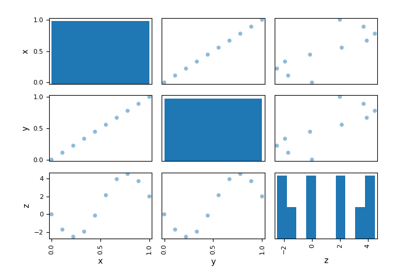Diagonal design of experiments

Diagonal design of experiments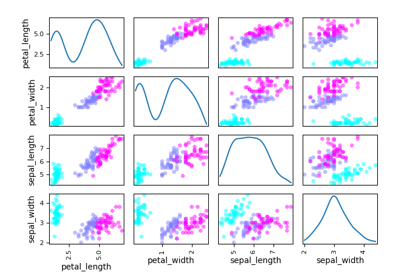Iris dataset

Iris dataset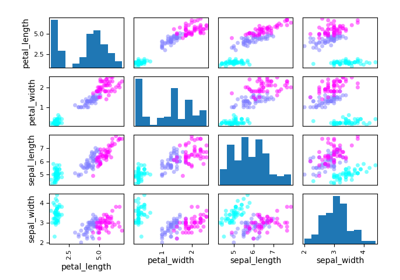Scatter matrix

Scatter matrix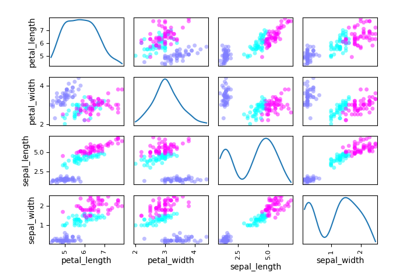Gaussian Mixtures

Gaussian Mixtures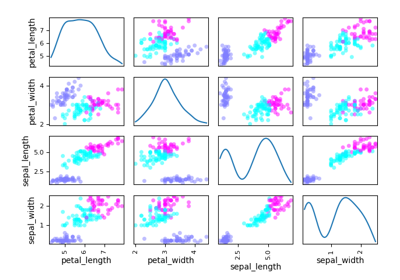K-means

K-means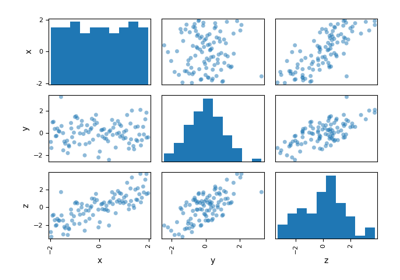Parameter space

Parameter spaceParameter space

Parameter space